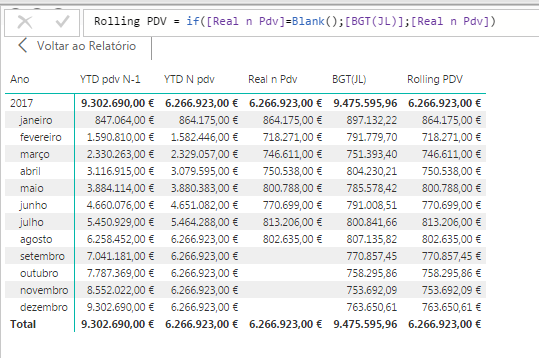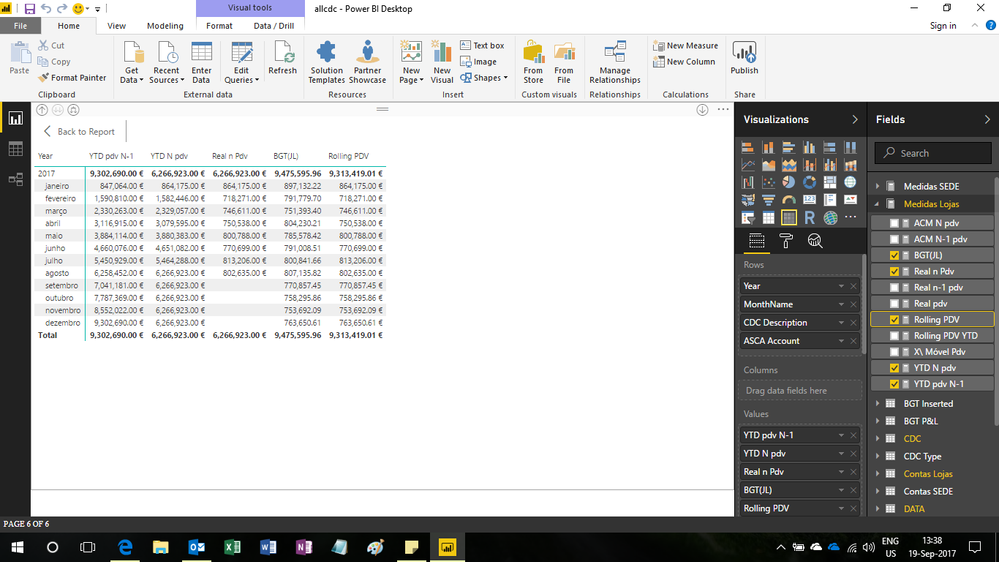cancel
Showing results for
Did you mean:Helper I

## Rolling = Real + BGT

Hello,

I´m trying to calculate only a a Real + Budget.

The formula is = If( [Real]<>Blank();[REal];[BGT]

It gives me month to month what i want.

The Problem is in the End ( The sum is Wrong). And if i make a YTD of it, it goes only to where i have Real Data..

Here is a Picture of it. Rooling PDV shoud be  = 9313K not 6.266K.1 ACCEPTED SOLUTIONSuper User

Hi,

I removed the data field from the row labels and dragged Year and Month Name field from the DATA Table.  I modified the Rolling PDV formula to

`=if(HASONEVALUE(DATA[MonthName]),if([Real n Pdv]=Blank(),[BGT(JL)],[Real n Pdv]),SUMX(SUMMARIZE(DATA,[MonthName],"ABCD",if([Real n Pdv]=Blank(),[BGT(JL)],[Real n Pdv])),[ABCD]))`

Here's a screenshotRegards,
Ashish Mathur
http://www.ashishmathur.com
11 REPLIES 11Super User

Hi,

your question is not clear.  What is 9313?  Where did that come from?

Regards,
Ashish Mathur
http://www.ashishmathur.comHelper I

9313K Should be the total of Rolling.

 864.175,00 718.271,00 746.611,00 750.538,00 800.788,00 770.699,00 813.206,00 802.635,00 770.857,00 758.295,00 753.692,00 763.650,00 9.313.417,00Super User

Hi,

Regards,
Ashish Mathur
http://www.ashishmathur.comHelper I

do you need the file?

How can i share the file?Super User

Regards,
Ashish Mathur
http://www.ashishmathur.comHelper ISuper User

Hi,

I removed the data field from the row labels and dragged Year and Month Name field from the DATA Table.  I modified the Rolling PDV formula to

`=if(HASONEVALUE(DATA[MonthName]),if([Real n Pdv]=Blank(),[BGT(JL)],[Real n Pdv]),SUMX(SUMMARIZE(DATA,[MonthName],"ABCD",if([Real n Pdv]=Blank(),[BGT(JL)],[Real n Pdv])),[ABCD]))`

Here's a screenshotRegards,
Ashish Mathur
http://www.ashishmathur.comHelper I

Thanks a lot!Super User

You are welcome.

Regards,
Ashish Mathur
http://www.ashishmathur.comFrequent Visitor

Hi,

excuse me but i don't have solution but i would like to create a message and how to post an image in a post (the insert image option input "source" ?

ThanksHelper I
Ctrl +printscreen . Save and post